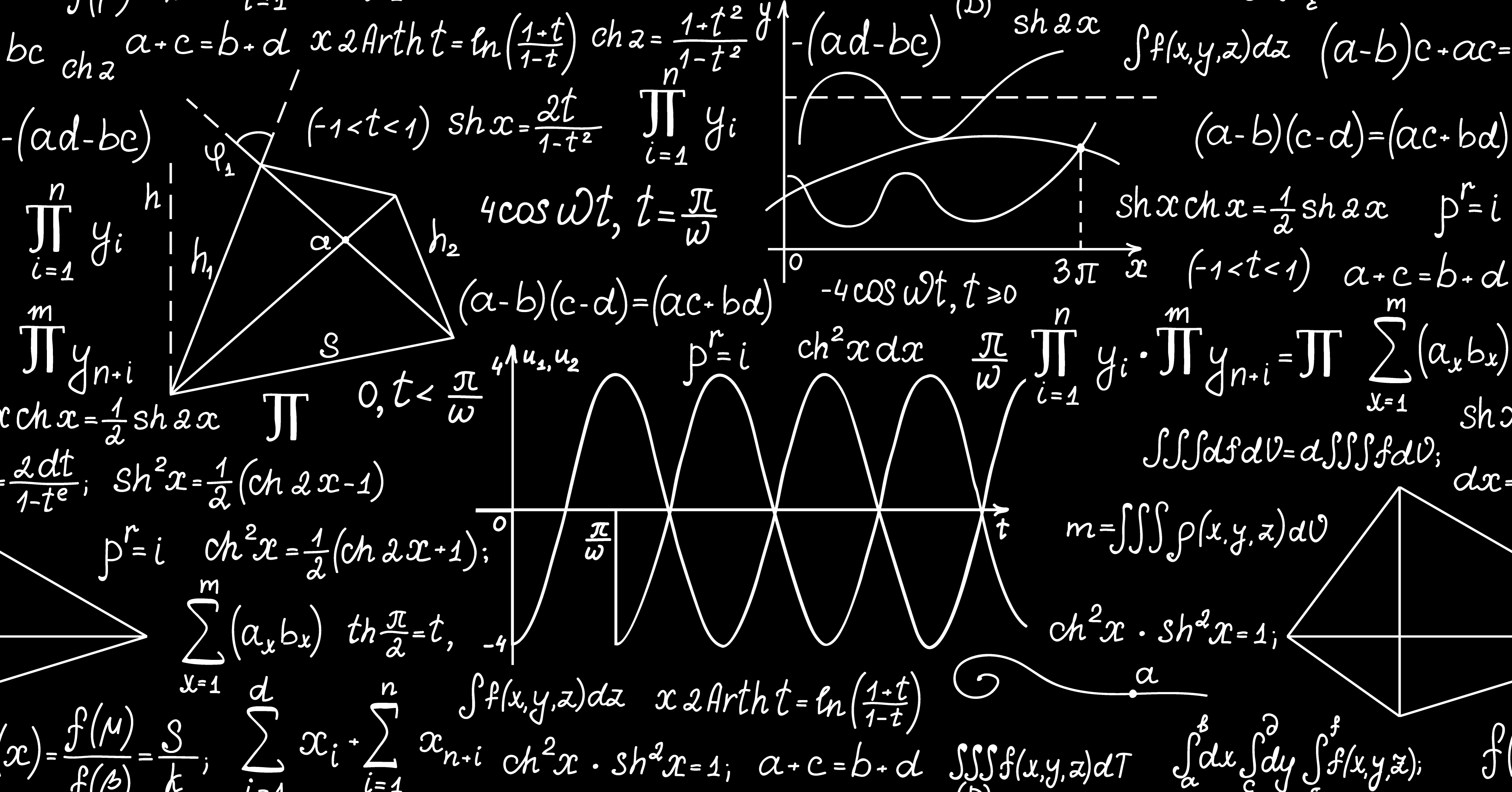# GMAT & MBA Admissions BlogThis post is the third in our series on using strategies to answer specific questions from the 2018 Official Guide. Here, one of our most experienced GMAT tutors, John Easter, analyzes a question about direct calculation.

125% of 5 =

(A) 5.125

(B) 5.25

(C) 6

(D) 6.125

(E) 6.25

When you're presented with a direct calculation, always start by scanning your answers. Clearly 125% of 5 is more than 5, but that doesn't help in this case. So, we've got two reasonable approaches, both of which rely to some extent on math facts.

1. Standard Operating Procedure: We like fractions better than decimals, and we know our fraction decimal equivalents (right???), so, 125% = 1.25 = 1 1/4 = 5/4. Now we just take the product 5(5/4) = 25/4 = 6 1/4 = 6.25.

2. Powers of 5: Learning your math facts is mostly about making friends. Sometime your friends can help you speed things up, but they can't help you unless you recognize them. This isn't as simple as it sounds because they appear in many contexts. This problem is an example of that. You have to look past the percent and realize that moving the decimal doesn't effect your math fact: 125 = 5^3 and 125(5) = 5^3(5) = 5^4 = 625... 6.25.